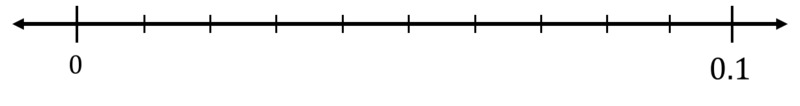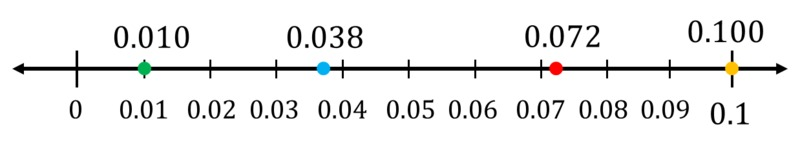# Placing Thousandths on the Number Line.

Alignments to Content Standards: 5.NBT.A.3

Label all of the tick marks on the number line.Plot and label each of the following numbers on the number line.

\begin{align} &0.100\\ &0.010\\ &0.072\\ &0.038 \end{align}

Which of these numbers is greatest? Which is least? How can you tell by looking at the number line?

## IM Commentary

Though this task primarily deals with comparing decimal numbers on a number line, it also requires students to draw upon what they know about the base ten system. They must recognize that the distance from 0 to 0.1 is partitioned into ten equal pieces and that one tenth of one tenth is 0.01. Once they realize that each increment goes up by 0.01, they can fill in the framework to place other numbers. One of the key instructional points in this task is that our number line orders numbers least to greatest. Therefore, numbers farther to the right are greater than those to the left. This understanding will hold true for future explorations of rational numbers as well. A good precursor task for this one would be 5.NBT Comparing Decimals on the Number Line. Side note: It would be good to have versions of this for thousandths that are not between 0 and 1.

## Solution0.010 is least because it is the farthest to the left on the number line and 0.100 is greatest because it is the farthest to the right on the number line.